# Learn Maths Full

1

별점

KOR• Numbers and Operation
• Powers and Exponents
• Number Line and Linear Inequalities
• Variables and Word Problems
• Polynomials
• Graphing Linear Equation
• Simultaneous Linear Equations
• Sets
• Linear Algebra
• Matrix Application
• Absolute Value
• And more contents about Calculus, Trigonometry, Statistics...

## 스크린샷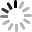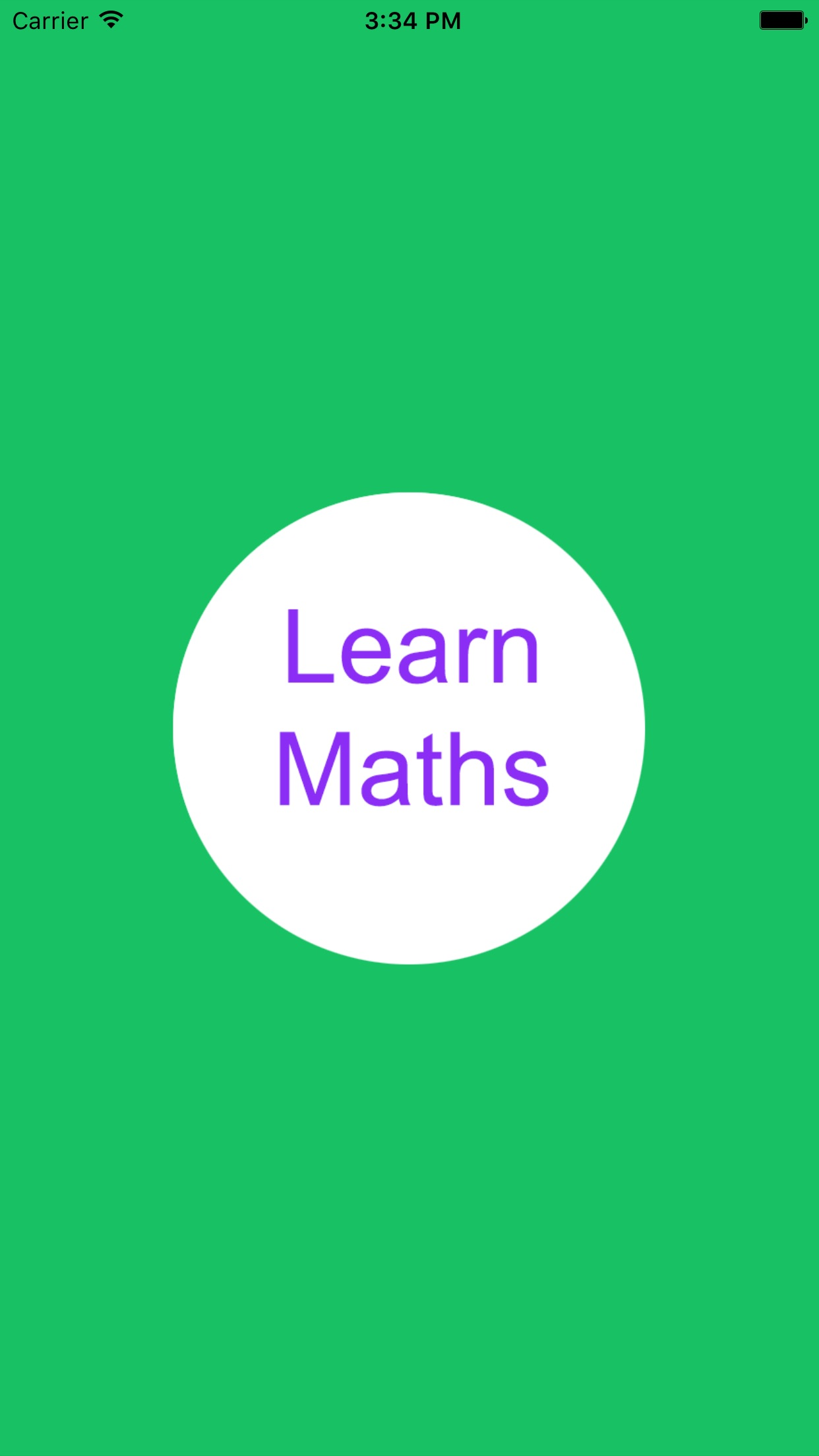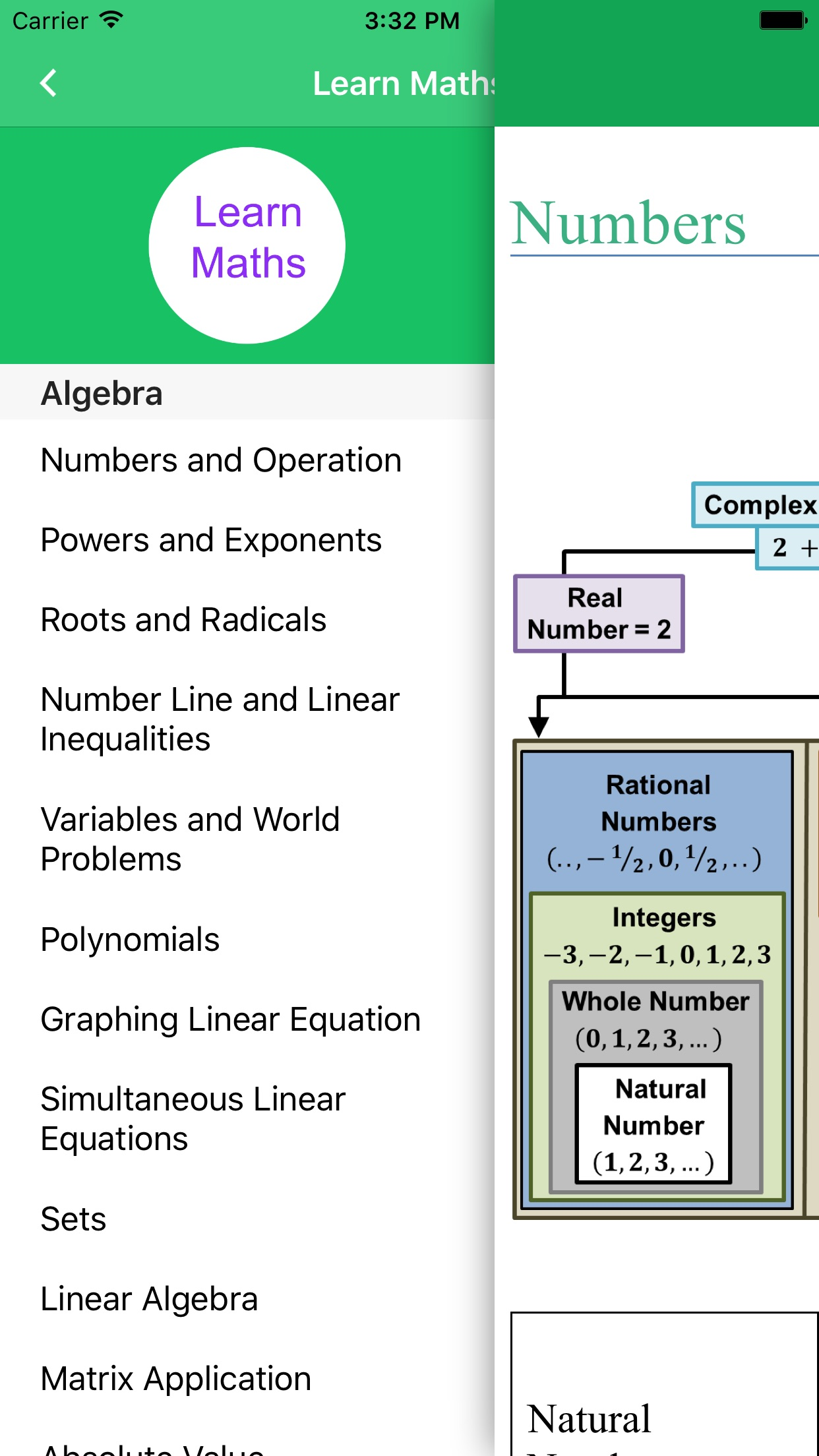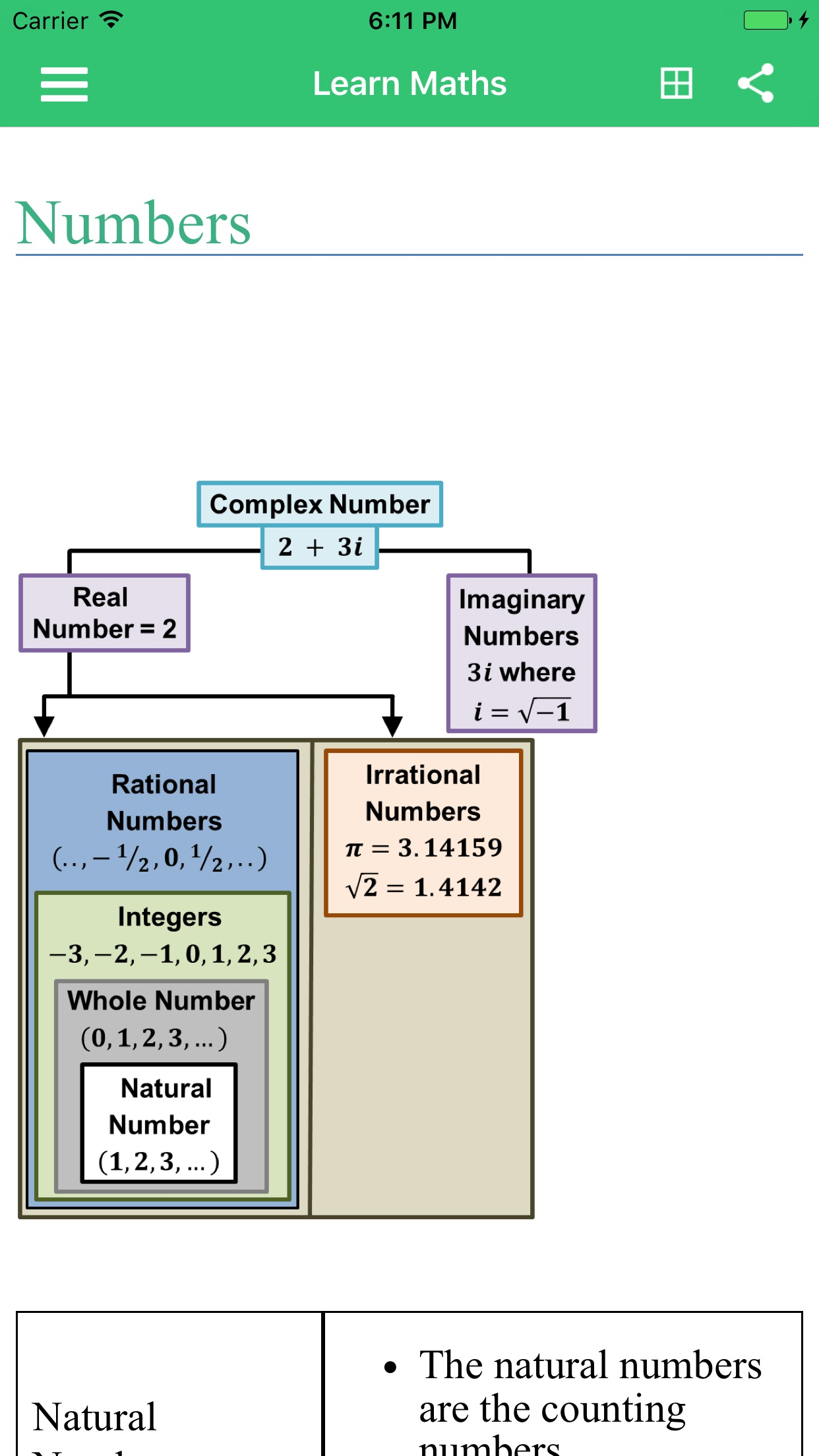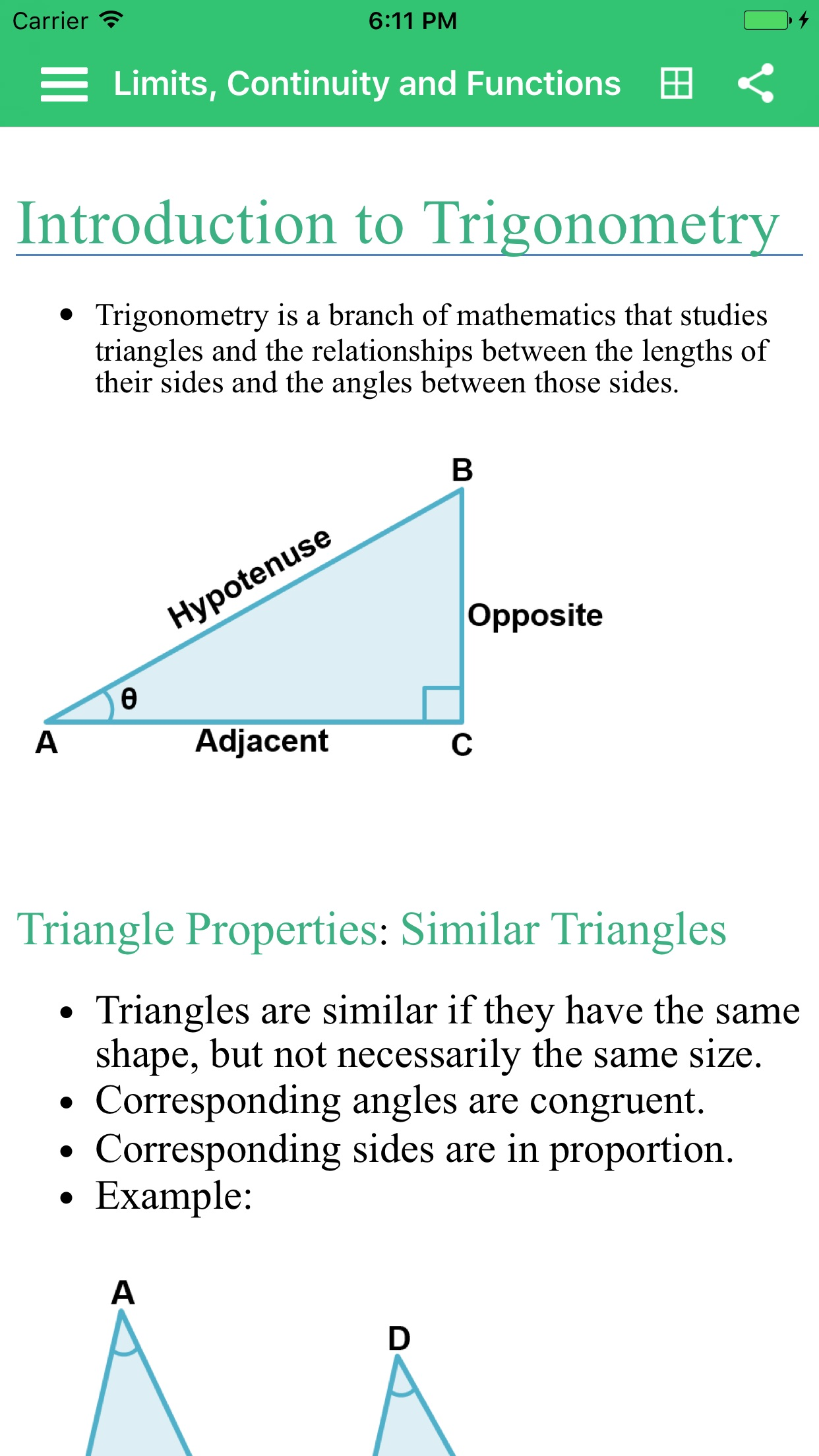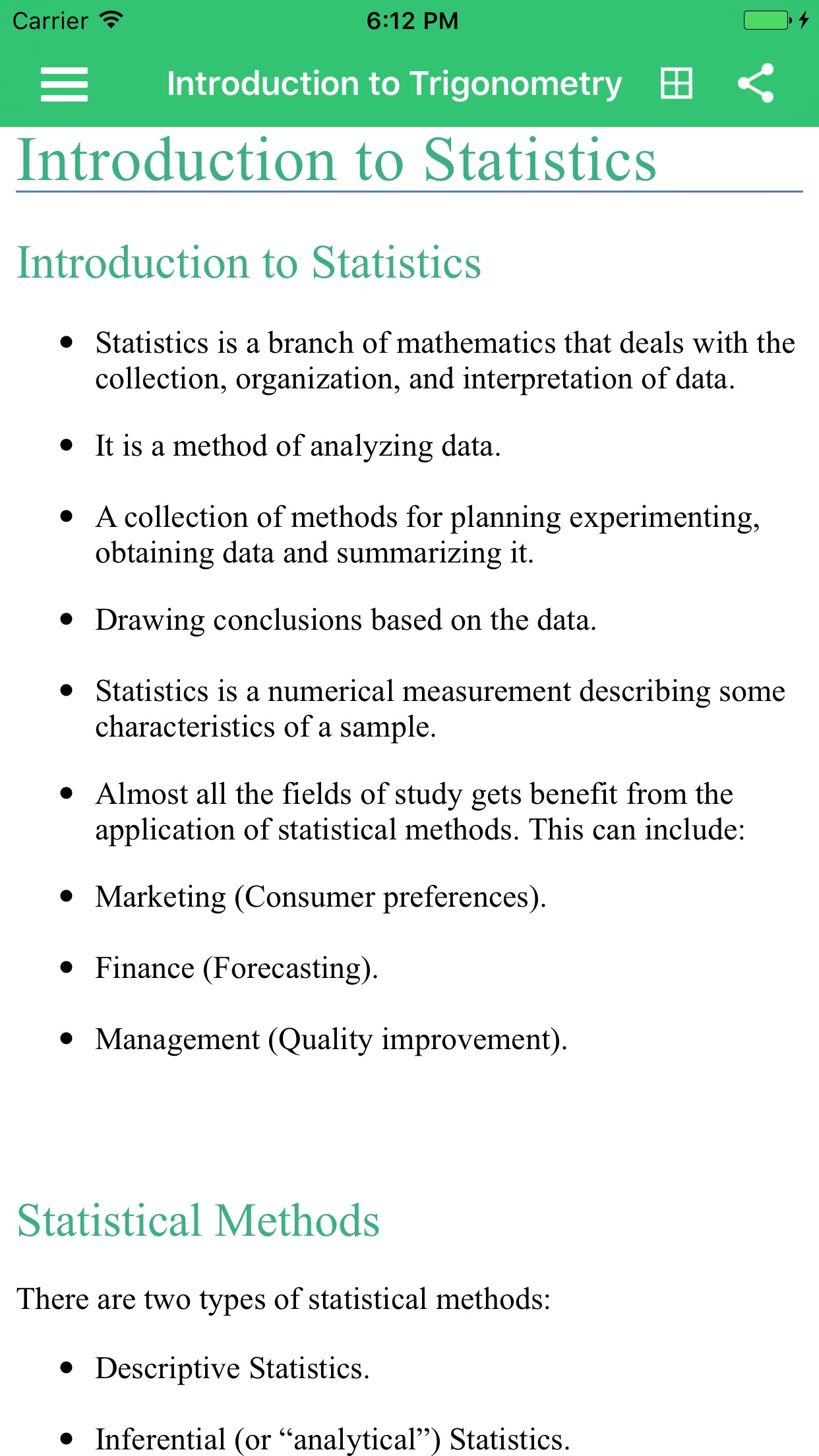• Android
• And Tab
• iPhone

0명 이상

### 앱 용량

• Version : 2.2
• 카테고리 :
• 개발사 : Woran Randoom
• 등록일 : 2016. 06. 07
정정신고

Woran Randoom